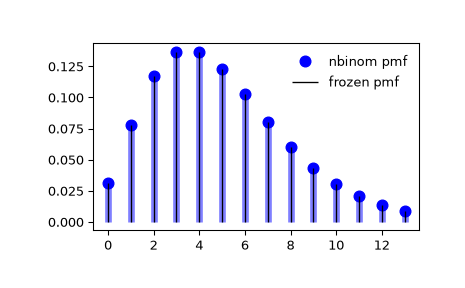# scipy.stats.nbinom¶

scipy.stats.nbinom(*args, **kwds) = <scipy.stats._discrete_distns.nbinom_gen object>[source]

A negative binomial discrete random variable.

As an instance of the rv_discrete class, nbinom object inherits from it a collection of generic methods (see below for the full list), and completes them with details specific for this particular distribution.

Notes

Negative binomial distribution describes a sequence of i.i.d. Bernoulli trials, repeated until a predefined, non-random number of successes occurs.

The probability mass function of the number of failures for nbinom is:

$f(k) = \binom{k+n-1}{n-1} p^n (1-p)^k$

for $$k \ge 0$$, $$0 < p \leq 1$$

nbinom takes $$n$$ and $$p$$ as shape parameters where n is the number of successes, $$p$$ is the probability of a single success, and $$1-p$$ is the probability of a single failure.

The probability mass function above is defined in the “standardized” form. To shift distribution use the loc parameter. Specifically, nbinom.pmf(k, n, p, loc) is identically equivalent to nbinom.pmf(k - loc, n, p).

Examples

>>> from scipy.stats import nbinom
>>> import matplotlib.pyplot as plt
>>> fig, ax = plt.subplots(1, 1)


Calculate a few first moments:

>>> n, p = 0.4, 0.4
>>> mean, var, skew, kurt = nbinom.stats(n, p, moments='mvsk')


Display the probability mass function (pmf):

>>> x = np.arange(nbinom.ppf(0.01, n, p),
...               nbinom.ppf(0.99, n, p))
>>> ax.plot(x, nbinom.pmf(x, n, p), 'bo', ms=8, label='nbinom pmf')
>>> ax.vlines(x, 0, nbinom.pmf(x, n, p), colors='b', lw=5, alpha=0.5)


Alternatively, the distribution object can be called (as a function) to fix the shape and location. This returns a “frozen” RV object holding the given parameters fixed.

Freeze the distribution and display the frozen pmf:

>>> rv = nbinom(n, p)
>>> ax.vlines(x, 0, rv.pmf(x), colors='k', linestyles='-', lw=1,
...         label='frozen pmf')
>>> ax.legend(loc='best', frameon=False)
>>> plt.show()Check accuracy of cdf and ppf:

>>> prob = nbinom.cdf(x, n, p)
>>> np.allclose(x, nbinom.ppf(prob, n, p))
True


Generate random numbers:

>>> r = nbinom.rvs(n, p, size=1000)


Methods

 rvs(n, p, loc=0, size=1, random_state=None) Random variates. pmf(k, n, p, loc=0) Probability mass function. logpmf(k, n, p, loc=0) Log of the probability mass function. cdf(k, n, p, loc=0) Cumulative distribution function. logcdf(k, n, p, loc=0) Log of the cumulative distribution function. sf(k, n, p, loc=0) Survival function (also defined as 1 - cdf, but sf is sometimes more accurate). logsf(k, n, p, loc=0) Log of the survival function. ppf(q, n, p, loc=0) Percent point function (inverse of cdf — percentiles). isf(q, n, p, loc=0) Inverse survival function (inverse of sf). stats(n, p, loc=0, moments=’mv’) Mean(‘m’), variance(‘v’), skew(‘s’), and/or kurtosis(‘k’). entropy(n, p, loc=0) (Differential) entropy of the RV. expect(func, args=(n, p), loc=0, lb=None, ub=None, conditional=False) Expected value of a function (of one argument) with respect to the distribution. median(n, p, loc=0) Median of the distribution. mean(n, p, loc=0) Mean of the distribution. var(n, p, loc=0) Variance of the distribution. std(n, p, loc=0) Standard deviation of the distribution. interval(alpha, n, p, loc=0) Endpoints of the range that contains alpha percent of the distribution

#### Previous topic

scipy.stats.logser

#### Next topic

scipy.stats.nhypergeom